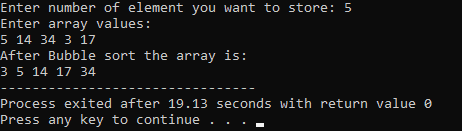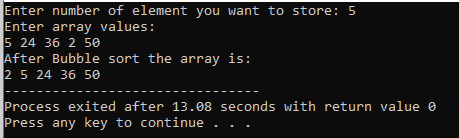# Bubble Sort In C++: The Complete Guide

C++ Bubble Sort is an algorithm that sorts the values of the array. Bubble Sort is a sorting technique to sort an array or sort a list of many numbers. The bubble sorting algorithm is also known as Sinking Sort.

We will implement the C++ Bubble sort program to demonstrate how we can use it in real-life applications. Although this is the simplest method to sort the list of numbers, it is not the best way. We will discuss it later.

In computer science, a sorting algorithm is an algorithm that puts items on the list in a particular order. Sorting is also often useful for canonicalizing data and for producing human-readable output.

Many sorting algorithms like Heap Sort, Bubble Sort, Merge Sort, Selection Sort, and Insertion Sort in C++. In this tutorial, we will discuss bubble sort and write a program of bubble sort step by step.

## How does bubble sort in C++ work?

In this sorting method, each element is compared with other list elements. Each time the largest, then the second-largest, then 3rd largest number is placed at its correct position, and so on until all the numbers are sorted. But, first, we will see one example of how it works?

### Example:

Suppose we are given a list of numbers: 15 5 23 2 17. Now, we will apply bubble sort in this example and will see how it works:

If we consider these numbers in an array, the array will be:

 0 1 2 3 4 Location 15 5 23 2 17 Array Value

So, when we apply bubble sort here, we will see how the array will change in each step.

### First Pass:

[15, 5 ,23 ,2 ,17] ———-> [5, 15, 23, 2 ,17]

[5, 15, 23, 2, 17] ———-> [5, 15, 23, 2, 17]

[5, 15, 23, 2, 17] ———-> [5, 15, 2, 23, 17]

[5, 15, 2, 23, 17] ———-> [5, 15, 2, 17, 23]

So, as said above, after every pass, the largest number will be placed at its correct place. Here, if you notice, you can see that a number is being compared with its adjacent number; if that number is larger than the next number (adjacent number), they are being swapped.

We 1st compared 15 and 5, as 15 is more significant than 5, so we have swapped them. Similarly, at the last step, 17 and 23 are compared, and then we have swapped them. So, the array finally became.

 Location 0 1 2 3 4 Array Value 5 15 2 17 23

Okay, now we will see the next passes:

### Second Pass:

[5, 15, 2, 17, 23] —————-> [5, 15, 2, 17, 23]

[5, 15, 2, 17, 23] —————-> [5, 2, 15, 17, 23]

[5, 2, 15, 17, 23] —————-> [5, 2, 15, 17, 23]

[5, 2, 15, 17, 23] —————-> [5, 2, 15, 17, 23]

So, after the second pass, we can see that only 2 are swapped with 15. Therefore, the rest are correct, although the pass continued until it reached the last number.

Now, after Second Pass, the array is:

 Location 0 1 2 3 4 Array Value 5 2 15 17 23

### Third Pass:

[5, 2, 15, 17, 23] ——————> [2, 5, 15, 17, 23]

[2, 5, 15, 17, 23] ——————> [2, 5, 15, 17, 23]

[2, 5, 15, 17, 23] ——————-> [2, 5, 15, 17, 23]

[2, 5, 15, 17, 23] ——————-> [2, 5, 15, 17, 23]

Now, you might be thinking that why I continued the steps after getting the sorted list? The answer is, we have two nested loops, so we have to continue the loop until the loop reaches its endpoint, no matter whether you got your sorted list or not.

Similarly, the Fourth Pass will also be executed without any reason:). But I am not going to show you that, as it will be boring.

Instead, we will see its algorithm.

## Algorithm for Bubble Sort in C++

Step1: Take the input of N numbers as an array arr

Step2: Repeat step 2 to 5 For(i=0;i<n;i++)

Step3: For(j=0;j<n-i-1;j++)

Step4: if(arr[j]>arr[j+1]

Step5: swap(arr[j],arr[j+1])

Step6: End

### Analysis:

In general, as we have N numbers, so we have to do

(N-1)+(N-2)+(N-3)+…+2+1 = ((N-1)*N)/2 comparisons.

So, the complexity of the algorithm is O(n^2)

This is for the worst-case and average-case.

The complexity for Best Case is O(n)

## Program of Bubble Sort in C++

```#include<bits/stdc++.h>
using namespace std;
int main()
{
int n;
cout<<"Enter number of element you want to store: ";
cin>>n;
int arr[n],i,j;
cout<<"Enter array values:\n";
//taking the array value
//from user
for(i=0;i<n;i++)
{
cin>>arr[i];
}
//Now we will sort the array
for(i=0;i<n-1;i++)
{
for(j=0;j<n-i-1;j++)
{
//checking if previous value is
//grater than next one or not
if(arr[j]>arr[j+1])
{
//temp will temporarly store
//the value of arr[j]
//then we will swap the values
int temp=arr[j];
arr[j]=arr[j+1];
arr[j+1]=temp;
}
}
}
cout<<"After Bubble sort the array is:\n";
for(i=0;i<n;i++)
cout<<arr[i]<<" ";
return 0;
}```

The output is above the program is following.Okay, now you might be thinking that we cannot optimize this program so that the loop gets over when we encounter a sorted list?

My answer is YES; we can. For this, please refer to the given program:

### Optimize solution of Bubble Sort:

Below is the optimized program.

```#include<bits/stdc++.h>
using namespace std;
int main()
{
int n;
cout<<"Enter number of element you want to store: ";
cin>>n;
int arr[n],i,j;
cout<<"Enter array values:\n";
//taking the array value
//from user
for(i=0;i<n;i++)
{
cin>>arr[i];
}
//here this flag will help
//to optimise the solution
//first initialise flag=1
int flag=1;
//Now we will sort the array
//if my flag value is 1 then only
//the loop will execute
for(i=0;i<n-1 && flag==1;i++)
{
//here after each time of j loop
// we will re-initialize the flag to 0
flag=0;
for(j=0;j<n-i-1;j++)
{
//checking if previous value is
//grater than next one or not
if(arr[j]>arr[j+1])
{
//temp will temporarly store
//the value of arr[j]
//then we will swap the values
int temp=arr[j];
arr[j]=arr[j+1];
arr[j+1]=temp;
//Here if there is a swap then
// we will make it 1
flag=1;
}
}
}
cout<<"After Bubble sort the array is:\n";
for(i=0;i<n;i++)
cout<<arr[i]<<" ";
return 0;
}```

The output of the above program is the following.That’s it for this tutorial.

## Recommended Posts

C++ Insertion Sort

C++ Heap Sort

C++ Quick Sort

C++ Merge Sort

C++ Selection Sort

Categories C++

This site uses Akismet to reduce spam. Learn how your comment data is processed.